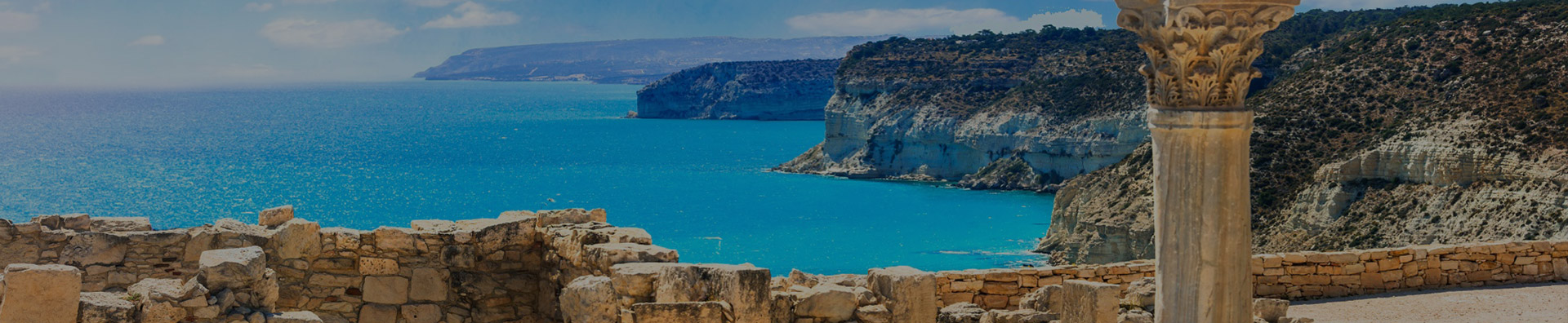# 利马索尔计划在海边建造“华尔街”

“将在本月底宣布将在滨海和新港口之间的新区域开始建造高层办公楼的计划–这个计划已经进行了四年。我们打算在这里建设类似纽约华尔街上这种类型的金融中心。我们将把银行，基金，外汇业务等集中在这一领域。

Tsouloftas解释说，为了使“华尔街”地区更具吸引力，该市计划增加在该地区将要建造的办公室的允许建筑系数。 “换句话说，根据建筑法规，特定土地上的建筑面积大小是有限制的。对于“华尔街”地区，此限制将大大提高，以便来自国外的公司可以实现其办公空间目标。”

Tsouloftas补充说，AKTEA大道将成为这个新金融区的主要动脉，将其与码头相连，并排成一排的棕榈树。

Tsouloftas继续说，技术园是另一个已搁置多年的项目，实际上将在今年内启动。

“这个项目是15年前开始的，但是在计划和发布招标中存在一些阻碍它的问题。该计划是在Pentakomo的海边使用一块土地-面积约54万平方米，属于政府所有。

Tsouloftas坚持认为，早日需要建立技术园区，因为利马索尔吸引了越来越多的外国科技公司，他们正在寻找这座城市的技术人才库和良好的基础设施。

“一旦塞浦路斯EastMed能源资源开发工作在大约一年后开始，利马索尔还将成为石油和天然气行业的中心。然后，将有大量的行业人士涌入我们的城市寻找办公室和房屋。有四家大型公司将致力于从该地区的资源中产生的项目。他们将需要在岸支持。

## 相关新闻### 请填写下表以联系我们

• 布基纳法索
• 沙特阿拉伯
• 土库曼斯坦
• 卡塔尔
• 尼泊尔
• 哈萨克斯坦
• 菲律宾
• 中国台湾
• 中国大陆
• 伊朗
• 哥斯达黎加
• 厄立特里亚
• 古巴
• 多米尼加共和国
• 墨西哥
• 尼加拉瓜
• 巴拿马
• 荷属安地列斯群岛
• 萨尔瓦多
• 英属维尔京群岛
• 波多黎各
• 美属维尔京群岛
• 圣多美和普林西比
• 安圭拉(英)
• 圣卢西亚
• 圣文森特和格纳丁斯
• 荷属安德列斯
• 瓜德罗普
• 洪都拉斯
• 危地马拉
• 格鲁吉亚
• 亚美尼亚
• 阿塞拜疆
• 安哥拉
• 白俄罗斯
• 俄罗斯联邦
• 乌克兰
• 欧洲其他国家(地区)
• 匈牙利
• 冰岛
• 马耳他
• 摩纳哥
• 挪威
• 罗马尼亚
• 利比亚
• 圣马力诺
• 瑞典
• 瑞士
• 爱沙尼亚
• 拉脱维亚
• 立陶宛
• 摩尔多瓦
• 土耳其
• 斯洛文尼亚
• 捷克共和国
• 非洲其他国家(地区)
• 斯洛伐克
• 马其顿
• 波斯尼亚-黑塞哥维那共和
• 梵蒂冈城国
• 荷兰
• 克罗地亚
• 希腊
• 爱尔兰
• 比利时
• 塞浦路斯
• 塞卜泰
• 丹麦
• 英国
• 德国
• 法国
• 意大利
• 卢森堡
• 葡萄牙
• 波兰
• 西班牙
• 阿尔巴尼亚
• 津巴布韦
• 安道尔
• 列支敦士登
• 塞黑
• 奥地利
• 保加利亚
• 芬兰
• 直布罗陀
• 多米尼克
• 百慕大
• 加拿大
• 几内亚
• 美国
• 格陵兰
• 北美洲其他国家(地区)
• 汤加
• 澳大利亚
• 库克群岛
• 瑙鲁
• 新喀里多尼亚
• 瓦努阿图
• 所罗门群岛
• 塞拉利昂
• 萨摩亚
• 图瓦卢
• 密克罗尼西亚联邦
• 马绍尔群岛
• 基里巴斯
• 法属玻利尼西亚
• 新西兰
• 斐济
• 巴布亚新几内亚
• 帕劳共和国
• 喀麦隆
• 留尼汪
• 土阿莫土群岛
• 土布艾群岛
• 社会群岛
• 马克萨斯群岛
• 瓦利斯和浮图纳
• 盖比群岛
• 诺福克岛
• 大洋洲其他国家(地区)
• 智利
• 哥伦比亚
• 加蓬
• 法属圭亚那
• 圭亚那
• 库腊索岛
• 巴拉圭
• 秘鲁
• 苏里南
• 委内瑞拉
• 乌拉圭
• 厄瓜多尔
• 安提瓜和巴布达
• 加纳
• 阿鲁巴岛
• 巴哈马
• 巴巴多斯
• 开曼群岛
• 格林纳达
• 萨巴
• 海地
• 牙买加
• 马提尼克
• 蒙特塞拉特
• 坦桑尼亚
• 圣文森特和格林纳丁斯
• 特立尼达和多巴哥
• 特克斯和凯科斯群岛
• 英属维尔京群岛
• 圣其茨-尼维斯
• 圣马丁岛
• 南美洲其他国家(地区)
• 圣皮埃尔和密克隆
• 阿根廷
• 伯利兹
• 马里
• 玻利维亚
• 巴西
• 博内尔
• 圣卢西亚
• 索马里
• 毛里塔尼亚
• 乌干达
• 乍得
• 马约特岛
• 贝宁
• 科摩罗
• 博茨瓦那
• 塞内加尔
• 斯威士兰
• 几内亚(比绍)
• 民主刚果
• 中非共和国
• 莱索托
• 刚果
• 南非
• 马达加斯加
• 利比里亚
• 突尼斯
• 赞比亚
• 尼日尔
• 西撒哈拉
• 多哥
• 纳米比亚
• 莫桑比克
• 梅利利亚
• 埃塞俄比亚
• 卢旺达
• 摩洛哥
• 马拉维
• 尼日利亚
• 佛得角
• 布隆迪
• 阿尔及利亚
• 吉布提
• 加那利群岛
• 冈比亚
• 赤道几内亚
• 塞舌尔
• 苏丹
• 肯尼亚
• 新加坡
• 韩国
• 叙利亚
• 乌兹别克斯坦
• 巴林
• 日本
• 约旦
• 越南
• 科特迪瓦
• 吉尔吉斯斯坦
• 泰国
• 斯里兰卡
• 阿联酋
• 老挝
• 阿富汗
• 中国澳门
• 塔吉克斯坦
• 朝鲜
• 巴勒斯坦
• 埃及
• 中国香港
• 伊拉克
• 黎巴嫩
• 科威特
• 文莱
• 马尔代夫
• 印度尼西亚
• 以色列
• 蒙古
• 亚洲其他国家(地区)
• 毛里求斯
• 阿曼
• 印度
• 缅甸
• 马来西亚
• 东帝汶
• 也门共和国
• 不丹
• 柬埔寨
• 巴基斯坦
• 孟加拉国×